Physics as a subject is vast and to have all the concepts of Physics compiled in a single place is indeed a boon to all students. The article has been tailored to meet the needs 10 + 2 or higher secondary students and for the students who are appearing for a competitive examination. It has everything a student would possibly need for their success in exams. The article attempts to consolidate important physics questions.

Q1) A 100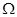resistor is connected to a 220 V, 50 Hz ac supply.

(a) What is the rms value of current in the circuit?

(b) What is the net power consumed over a full cycle?

Ans: Resistance of the resistor, R = 100Supply voltage, V = 220 V

Frequency,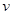= 50 Hz

(a) The rms value of current in the circuit is given as: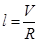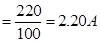(b) The net power consumed over a full cycle is given as:

P = VI

= 220 × 2.2 = 484 W

Q2) (a) The peak voltage of an ac supply is 300 V. What is the rms voltage?

(b) The rms value of current in an ac circuit is 10 A. What is the peak current?

Ans: (a) Peak voltage of the ac supply,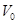= 300 V

Rms voltage is given as: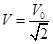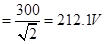(b) The rms value of current is given as:

I = 10 A

Now, peak current is given as: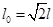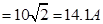Q3) A 44 mH inductor is connected to 220 V, 50 Hz ac supply. Determine the rms value of the current in the circuit.

Ans: Inductance of inductor, L = 44 mH =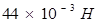Supply voltage, V = 220 V

Frequency,= 50 Hz

Angular frequency,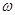=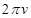Inductive reactance, XL =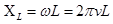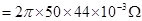Rms value of current is given as: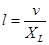s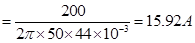Hence, the rms value of current in the circuit is 15.92 A.

Q4) A 60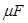capacitor is connected to a 110 V, 60 Hz ac supply. Determine the rms value of the current in the circuit.

Ans: Capacitance of capacitor, C = 60=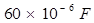Supply voltage, V = 110 V

Frequency,= 60 Hz

Angular frequency,=Capacitive reactance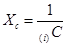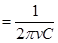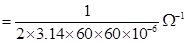Rms value of current is given as: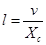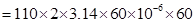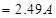Hence, the rms value of current is 2.49 A.

Q5) Choose the correct alternative from the clues given at the end of the each statement:

(a) The size of the atom in Thomson’s model is ………. the atomic size in Rutherford’s model. (much greater than/no different from/much less than.)

(b) In the ground state of ………. electrons are in stable equilibrium, while in ………. electrons always experience a net force.

(Thomson’s model/ Rutherford’s model.)

(c) A classical atom based on ………. is doomed to collapse.

(Thomson’s model/ Rutherford’s model.)

(d) An atom has a nearly continuous mass distribution in a ………. but has a highly non-uniform mass distribution in ……….

(Thomson’s model/ Rutherford’s model.)

(e) The positively charged part of the atom possesses most of the mass in ………. (Rutherford’s model/both the models.)

Ans: (a) The sizes of the atoms taken in Thomson’s model and Rutherford’s model have the same order of magnitude.

(b) In the ground state of Thomson’s model, the electrons are in stable equilibrium. However, in Rutherford’s model, the electrons always experience a net force.

(c) A classical atom based on Rutherford’s model is doomed to collapse.

(d) An atom has a nearly continuous mass distribution in Thomson’s model, but has a highly non-uniform mass distribution in Rutherford’s model.

(e) The positively charged part of the atom possesses most of the mass in both the models.

Q6) Suppose you are given a chance to repeat the alpha-particle scattering experiment using a thin sheet of solid hydrogen in place of the gold foil. (Hydrogen is a solid at temperatures below 14 K.) What results do you expect?

Ans: In the alpha-particle scattering experiment, if a thin sheet of solid hydrogen is used in place of a gold foil, then the scattering angle would not be large enough. This is because the mass of hydrogen (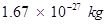) is less than the mass of incident α-particles (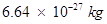). Thus, the mass of the scattering particle is more than the target nucleus (hydrogen). As a result, the α-particles would not bounce back if solid hydrogen is used in the α-particle scattering experiment.

Q7) The storage battery of a car has an emf of 12 V. If the internal resistance of the battery is 0.4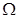, what is the maximum current that can be drawn from the battery?

Ans: Emf of the battery, = 12 V

Internal resistance of the battery, r = 0.4 Ω

Maximum current drawn from the battery can be calculated as:

The maximum current drawn from the given battery is 30 A.

Q8) A battery of emf 10 V and internal resistance 3is connected resistor. If the current in the circuit is 0.5 A, what is the resistance of the resistor? What is the terminal voltage of the battery when the circuit is closed?

Ans: Emf of the battery, E=10V

Internal resistance of the battery ,r =3Current in the circuit, I=0.5A

Resistance of the resistor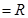The relation for current using Ohm’s law is,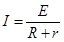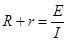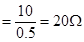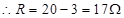Terminal voltage of the resistor=V

According to Ohm’s law,

V=IR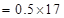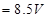Therefore, the resistance of the resistor is 17and the terminal voltage is 8.5v.

Q9)  Is it necessary for a transmitting antenna to be at the same height as that of the receiving antenna for line-of-sight communication? A TV transmitting antenna is 81m tall. How much service area can it cover if the receiving antenna is at the ground level?

Ans: Line-of-sight communication means that there is no physical obstruction between the transmitter and the receiver. In such communications it is not necessary for the transmitting and receiving antennas to be at the same height.

Height of the given antenna, h = 81 m

Radius of earth, R =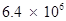m

For range, d = (2Rh)½, the service area of the antenna is given by the relation:

A =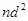=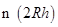=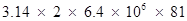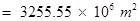= 3255.55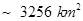Q10)  Frequencies in the UHF range normally propagate by means of:

(a) Ground waves.

(b) Sky waves.

(c) Surface waves.

(d) Space waves.

Ans: (d) Space waves

Owing to its high frequency, an ultra high frequency (UHF)wave can neither travel along the trajectory of the ground nor can it get reflected by the ionosphere. The signals having UHF are propagated through line-of-sight communication, which is nothing but space wave propagation.

Q11) A long straight wire in the horizontal plane carries a current of 50 A in north to south direction. Give the magnitude and direction of B at a point 2.5 m east of the wire.

Ans: Current in the wire, I = 50 A

A point is 2.5 m away from the East of the wire.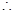Magnitude of the distance of the point from the wire, r = 2.5 m.

Magnitude of the magnetic field at that point is given by the relation, B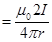Where,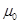= Permeability of free space =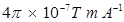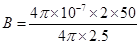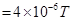The point is located normal to the wire length at a distance of 2.5 m. The direction of the current in the wire is vertically downward. Hence, according to the Maxwell’s right hand thumb rule, the direction of the magnetic field at the given point is vertically upward.

Q12) For sound waves, the Doppler formula for frequency shift differs slightly between the two situations: (i) source at rest; observer moving, and (ii) source moving; observer at rest. The exact Doppler formulas for the case of light waves in vacuum are, however, strictly identical for these situations. Explain why this should be so. Would you expect the formulas to be strictly identical for the two situations in case of light travelling in a medium?

Ans: No Sound waves can propagate only through a medium. The two given situations are not scientifically identical because the motion of an observer relative to a medium is different in the two situations. Hence, the Doppler formulas for the two situations cannot be the same.

In case of light waves, sound can travel in a vacuum. In a vacuum, the above two cases are identical because the speed of light is independent of the motion of the observer and the motion of the source. When light travels in a medium, the above two cases are not identical because the speed of light depends on the wavelength of the medium.

Q13) Answer the following questions: (a) In a single slit diffraction experiment, the width of the slit is made double the original width. How does this affect the size and intensity of the central diffraction band?

(b) In what way is diffraction from each slit related to the interference pattern in a double-slit experiment?

(c) When a tiny circular obstacle is placed in the path of light from a distant source, a bright spot is seen at the centre of the shadow of the obstacle. Explain why?

(d) Two students are separated by a 7 m partition wall in a room 10 m high. If both light and sound waves can bend around obstacles, how is it that the students are unable to see each other even though they can converse easily.

(e) Ray optics is based on the assumption that light travels in a straight line. Diffraction effects (observed when light propagates through small apertures/slits or around small obstacles) disprove this assumption. Yet the ray optics assumption is so commonly used in understanding location and several other properties of images in optical instruments. What is the justification?

Ans: (a) In a single slit diffraction experiment, if the width of the slit is made double the original width, then the size of the central diffraction band reduces to half and the intensity of the central diffraction band increases up to four times.

(b) The interference pattern in a double-slit experiment is modulated by diffraction from each slit. The pattern is the result of the interference of the diffracted wave from each slit.

(c) When a tiny circular obstacle is placed in the path of light from a distant source, a bright spot is seen at the centre of the shadow of the obstacle. This is because light waves are diffracted from the edge of the circular obstacle, which interferes constructively at the centre of the shadow. This constructive interference produces a bright spot.

(d) Bending of waves by obstacles by a large angle is possible when the size of the obstacle is comparable to the wavelength of the waves.

On the one hand, the wavelength of the light waves is too small in comparison to the size of the obstacle. Thus, the diffraction angle will be very small. Hence, the students are unable to see each other. On the other hand, the size of the wall is comparable to the wavelength of the sound waves. Thus, the bending of the waves takes place at a large angle. Hence, the students are able to hear each other.

(e) The justification is that in ordinary optical instruments, the size of the aperture involved is much larger than the wavelength of the light used.

Q14)The normal activity of living carbon-containing matter is found to be about 15 decays per minute for every gram of carbon. This activity arises from the small proportion of radioactive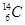present with the stable carbon isotope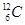. When the organism is dead, its interaction with the atmosphere (which maintains the above equilibrium activity) ceases and its activity begins to drop. From the known half-life (5730 years) of, and the measured activity, the age of the specimen can be approximately estimated. This is the principle ofdating used in archaeology. Suppose a specimen from Mohenjodaro gives an activity of 9 decays per minute per gram of carbon. Estimate the approximate age of the Indus-Valley civilisation.

Ans: Decay rate of living carbon-containing matter, R= 15 decay/min

Let N be the number of radioactive atoms present in a normal carbon- containing matter.

Half life of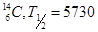years,

The decay rate of the specimen obtained from the Mohenjodaro site:

R‘ = 9 decays/min

Let N’ be the number of radioactive atoms present in the specimen during the Mohenjodaro period.

Therefore, we can relate the decay constant,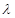and time, t as: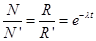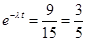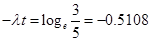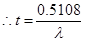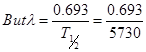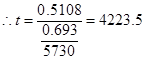Hence, the approximate age of the Indus-Valley civilisation is 4223.5 years.

Also check our article on Physics notes for Class 12 here.

## Mock questions are too easy or too hard?

We adapt to your current skill level and rapidly raise it up.

+91
No thanks.

## Request a Free 60 minute counselling session at your home

Please enter a valid phone number
•7,829,648

Happy Students
•358,177,393

Questions Attempted
•3,028,498

Tests Taken
•3,020,367